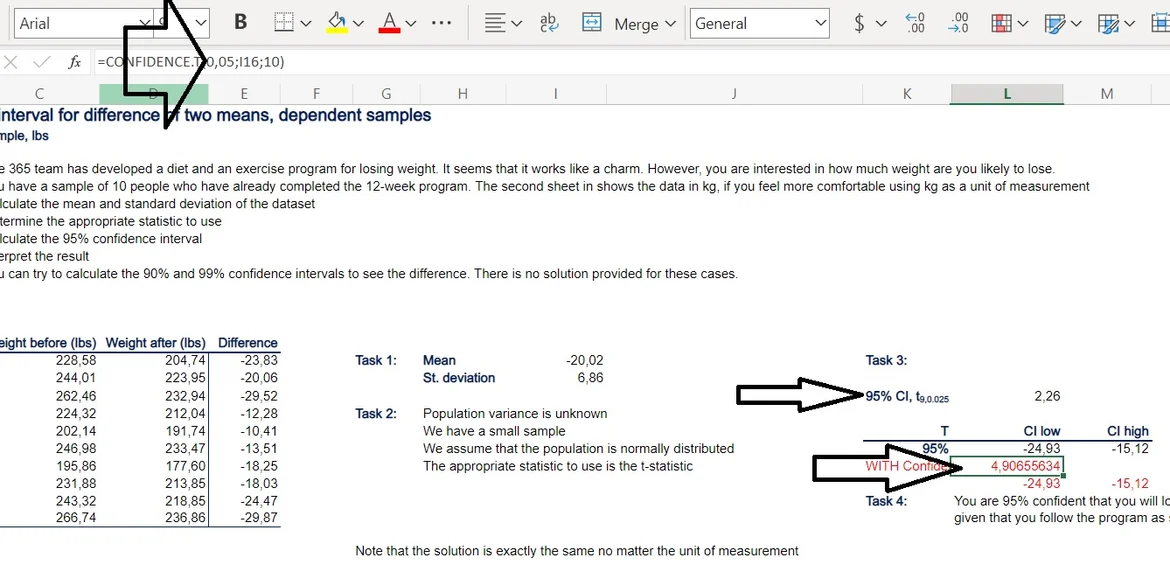14 Nov 2022

Posted on:

11 Nov 2022

1

# Resolved:Using Excel built-in CONFIDENCE() function to find the intervals

Hello, I would like to ask some question. For this exercise I have tried the way that's being taught in this course which is to calculate Mean, StDev, Standard Error, finding the z/t value through the table and then chucking the number in the formula. I've arrived at the same answer that's being provided in the "-solution" file. But I'm wondering and been experimenting using the CONFIDENCE function of excel that just need the arguments of (alpha, stdev, size) and then just add/substract the result value with the mean. By going this route I've arrived at an answer that's close to the previous methods that's being taught but not the exact same. Is there something that might be wrong from my own input? I want to know what makes the different answer even if the underlying concept is the same

Instructor
Posted on:

11 Nov 2022

0

Hi,
Hope you're well.
Is the Excel function considering whether you use population or sample data?
Best,
Ned

Posted on:

11 Nov 2022

0

The excel function just asks for standard deviation and I do properly input it using STDEV.P() and STDEV.S() for Population and Sample respectively depending on the context. The difference in results is miniscule. For example, in exercise 4.2 by using CONFIDENCE method then adding to and subtract from the mean I get results 95,696-104,705 whereas using the methods that's explained in this course get me the exact amount like in the "-solution" file which is 95,682-104,719. Since the practice case for exercise 4.2 using data about salary this raise some concern for me cause even though the difference is miniscule it still needs to be exact because of the context. Is there might be some hint or solution to resolve this problem? Because ultimately it will be helpful if there are more methods to tackle one problem.

Posted on:

14 Nov 2022

0

Hi, I have also used confidence. The difference is, that alfa is not /2